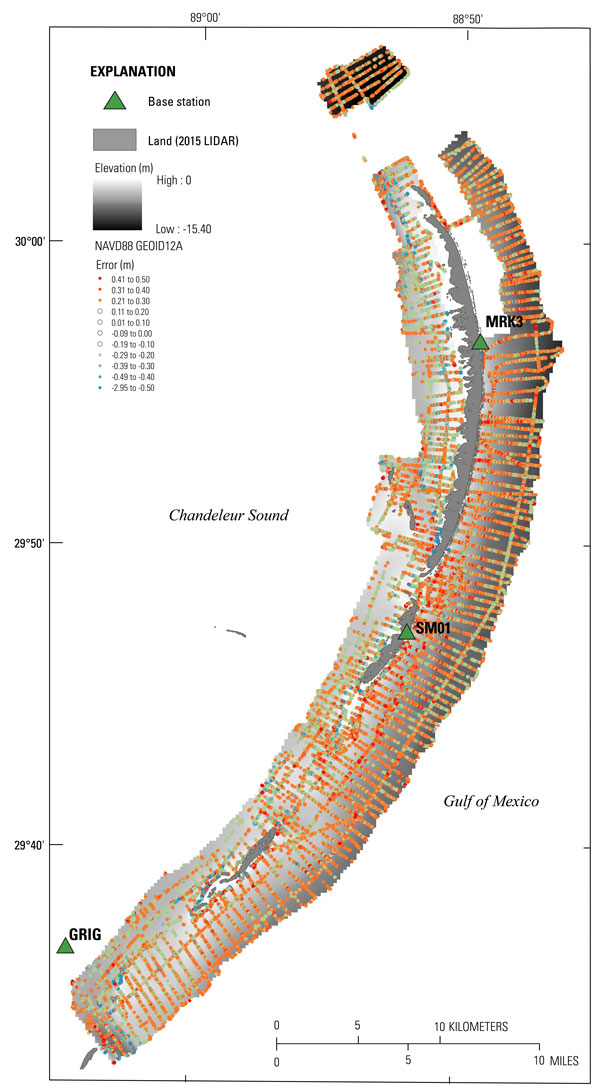Data Series 1039

### Coastal Single-Beam Bathymetry Data Collected in 2015 from the Chandeleur Islands, Louisiana

 Citation Page Abstract Introduction Survey Overview Data Acquisition Data Processing Error Analysis Survey Products Data Downloads Logs Abbreviations References Cited

## Error Analysis

### Digital Elevation Model (DEM) Error

In order to evaluate how well the DEM represents the final sounding data, a comparison of the DEM cell value versus the final point elevations were plotted in ArcGIS using the “extract values by points” spatial analyst tool. This tool extracts the value represented by the underlying grid and compares it to that of the overlying point data. The root mean square (RMS) error, quantified as the difference between the measured depth and the interpolated depth values, was calculated for each platform using the following equation, where “X” represents the data points, and “n” is the number of samples represented by the grid:There are a total of 7,556,180 point elevations represented by the grid, and the RMS error of all points is 0.23 m. Data that falls between -0.2 and 0.2 m difference error contributes to 74 percent of the data and has an RMS of 0.10 m. To represent the spatial distribution of grid error, the difference between the DEM and the original sounding values were plotted, color coded, and overlaid on the DEM (fig. 8).Figure 8. Error values representing the difference between the Digital Elevation Model (DEM) and the value at each sample point. Points with acceptable grid error of +0.20 to -0.19 meters (m) were made transparent to ensure visibility of points and areas that are greater in error. [Click figure to enlarge]U.S. Department of the Interior | U.S. Geological Survey
URL: https://pubs.usgs.gov/ds/1039/ds1039_error-analysis.html